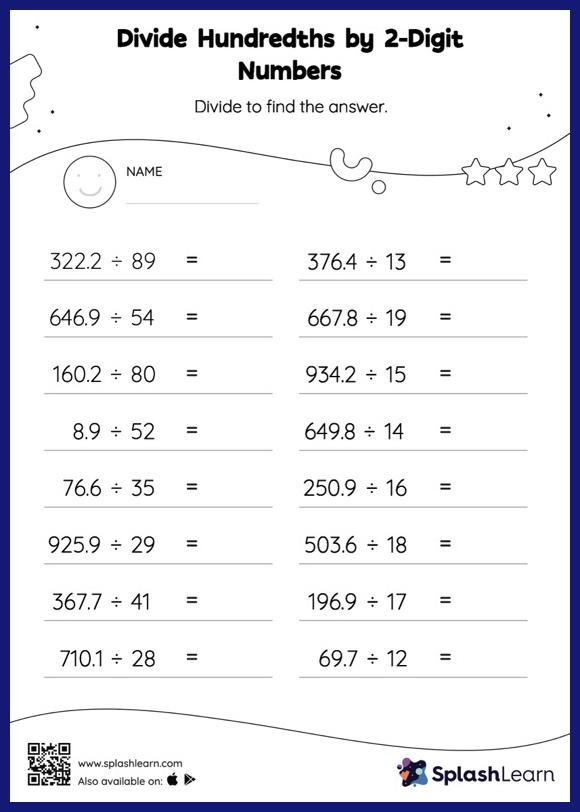# Divide Hundredths by 2-Digit Numbers with Remainder: Horizontal Division Worksheet

Home > Divide Hundredths by 2-Digit Numbers with Remainder: Horizontal DivisionDoes your child know how to divide hundredths by 2-digit numbers with remainder? Invite them to practice this concept here. Students divide decimals by 2-digit numbers in the same manner as they would divide whole numbers first. Depending on how many decimal digits are in the dividend, they add a decimal point at the appropriate place in the quotient. Their accuracy with this concept is improved by using the divide hundredths by 2-digit numbers with remainder worksheet.